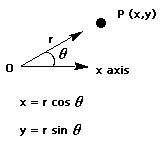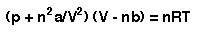## Problem Set

### Write three programs

The problems are divided into two sets:
you must complete at least one from the more difficult set.

Exam Mark Printer

Write a program to take in a set of ten exam marks from the keyboard, sort them into increasing numerical order and print them out. The program should print the student number (assume the marks are entered in order of increasing student number, so the first mark belongs to student 1, etc.) alongside the exam mark. The program should produce the output as a printed list.

Stirling's Approximation

logN! = NlogN-N

is Stirling's approximation, used in statistical thermo-dynamics. Write a program to check this formula for N=10, N=100, N=1000 and N=5000, bearing in mind that 5000! is much too large a number to store in the computer.

Fibonacci Series

The fibonacci series of numbers proceeds as follows 1, 1, 2, 3, 5, 8, 13..... Calculate the first 25 terms and store them in an array, print out the contents of the array.

Polar Coordinates

One set of polar coordinates of a point is given by (r, q), where r is the length of the ray from the origin to the point and q is the measure of an angle from the positive x axis to this ray.Write a program that reads polar coordinates for a point (in distance and degrees -not radians) and calculates and displays its rectangular coordinates (x,y) obtained by using the formulas above.

Van der Waals Equation

The van der Waals equation for gases is an extension of the equation PV=nRT which attempts to take into account the deviation of real gases from ideal behaviour. The equation is:in which n is the number of moles of gas, p its pressure (Pa), T the temperature (K), V the volume (m ), R the gas constant (8.314 J mol-l K-l) and a and b are constants which measure the deviation from ideality of the gas behaviour. Rearrange this equation so that it gives p as a function of V, then write a program that prints a table with p for 10 volumes between Vl and V2. The variables a, b, T, Vl,V2 and n should be entered from the keyboard. For Hydrogen a=0.0247 Pa m6mol-2 and b=26.6x10-6 m3mol-l. For CarbonDioxide a=0.366 Pa m6mol-2 and b=42.9x10-6 m3mol-l.